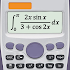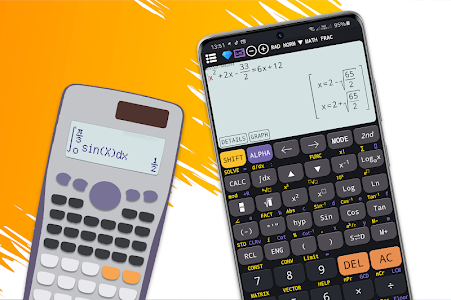# Free scientific calculator plus advanced 991 calc v5.2.9.702

Free

### Free scientific calculator plus advanced 991 calc / Screenshots### Free scientific calculator plus advanced 991 calc / Description

Scientific calculator 300 plus 991 is the calculator for students and engineering student. Calculator 991 plus provides powerful functions in a real calculator 991 300 . It makes advanced calculator 991 become the most useful calculator for university and school.

Application includes some calculators inside:
- Basic calculator 300 plus
- Scientific calculator 991
- Scientific calculator 991 plus
- Graphing calculator 84 plus

Let's see some special features:

◉ Basic calculator 300 plus and 115 plus: supports from basic mathematics to advanced math functions: percentage powers roots trigonometric logarithms calculator. Fraction calculation 991 supports convert fraction to decimal mixed fraction and solve fractions problems.

◉ Advanced calculator 115 plus: combines of hyper calculator and simple scientific calc. This calculator is a multi-functional advanced calculator 991 with all features in one app. Such as linear algebra calculus complex numbers display result in rectangular and polar coordinates matrix and vector.

◉ Scientific calculator 991 plus: includes smart equation solver. Equation solver can solve quadratic cubic equations systems of equations. Caluculator solves any polynomial. Smart scientific calculator 991 has exponent calculator contains scientific calculations such as derivative integral square root calculation factorial calculation pi calculation equation solver.

◉ Scientific calculator 991: supports calculate with infinity number of digits prime factors random numbers combinations permutations GCD and LCM. This calculator 991ex is a real engineering simulator. Scientific calculator has features of 500 es 500 ms 300 es plus 991 es plus. Calculator keyboard layout same as calculator 300 es plus 991 ex and es plus.

◉ Graphing calculator 84 plus supports function graph polar parametric and implicit function. Graphing calculator 84 can draw tangent tracing derivative root min and max. It simulates for graphing caluculator 83 and t1 84 84 plus.

◉ Integral calculator 991 plus provide powerful calculator to calculate integrate derivative differentiation and integration.

◉ This scientific calculator 991 includes CAS (computer algebra system) which can perform symbolic calculation.

◉ Some other features: math formula physics formula unit conversion theme font and programming.